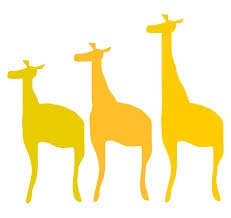# Variation Variety

Algebra Level 2x varies directly with y, and inversely with $z^{2}$. When y = 2 and
z = 3, x equals 8. What does x equal when y = 5 and z = 6?

×